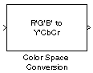# Color Space Conversion

Convert color information between color spaces

• Library:
• Computer Vision Toolbox / Conversions

•## Description

The Color Space Conversion block converts color information between color spaces. Use the Conversion parameter to specify the color spaces you are converting between.

• `R'G'B' to Y'CbCr`

• `Y'CbCr to R'G'B'`

• `R'G'B' to intensity`

• `R'G'B' to HSV`

• `HSV to R'G'B'`

• `sR'G'B' to XYZ`

• `XYZ to sR'G'B'`

• `sR'G'B' to L*a*b*`

• `L*a*b* to sR'G'B'`

## Ports

### Input

expand all

Input color information, specified as an M-by-N-by-P color matrix of P color planes.

Data Types: `single` | `double` | `int8` | `uint8`

R plane of the RGB color information, specified as an M-by-N matrix.

Data Types: `single` | `double` | `int8` | `uint8`

G plane of the RGB color information, specified as an M-by-N matrix.

Data Types: `single` | `double` | `int8` | `uint8`

B plane of the RGB color information, specified as an M-by-N matrix.

Data Types: `single` | `double` | `int8` | `uint8`

Luma signal component, specified as an M-by-N matrix.

Data Types: `single` | `double` | `int8` | `uint8`

Chrominance component, specified as an M-by-N matrix.

Data Types: `single` | `double` | `int8` | `uint8`

Chrominance component, specified as an M-by-N matrix.

Data Types: `single` | `double` | `int8` | `uint8`

Intensity values specified as an M-by-N matrix.

Data Types: `single` | `double` | `int8` | `uint8`

Hue component, specified as an M-by-N matrix.

Data Types: `single` | `double`

Saturation component, specified as an M-by-N matrix.

Data Types: `single` | `double`

Brightness component, specified as an M-by-N matrix.

Data Types: `single` | `double`

X component, specified as an M-by-N matrix.

Data Types: `single` | `double`

Y component, specified as an M-by-N matrix.

Data Types: `single` | `double`

Z component, specified as an M-by-N matrix.

Data Types: `single` | `double`

Luminance component, specified as an M-by-N matrix.

Data Types: `single` | `double`

a* component, specified as an M-by-N matrix.

Data Types: `single` | `double`

b* component, specified as an M-by-N matrix.

Data Types: `single` | `double`

### Output

expand all

Input color information, specified as an M-by-N-by-P color matrix of P color planes.

• If the input is `uint8`, then `Y'CbCr` is `uint8`, where `Y` is in the range `[16 235]`, and `Cb` and `Cr` are in the range `[16 240]`.

• If the input is a `double`, then `Y'` is in the range `[16/255 235/255]` and `Cb` and `Cr` are in the range ```[16/255 240/255]```.

Data Types: `single` | `double` | `int8` | `uint8`

R plane of the RGB color information, specified as an M-by-N matrix.

Data Types: `single` | `double` | `int8` | `uint8`

G plane of the RGB color information, specified as an M-by-N matrix.

Data Types: `single` | `double` | `int8` | `uint8`

B plane of the RGB color information, specified as an M-by-N matrix.

Data Types: `single` | `double` | `int8` | `uint8`

Luma signal component, specified as an M-by-N matrix.

Data Types: `single` | `double` | `int8` | `uint8`

Chrominance component, specified as an M-by-N matrix.

Data Types: `single` | `double` | `int8` | `uint8`

Chrominance component, specified as an M-by-N matrix.

Data Types: `single` | `double` | `int8` | `uint8`

Intensity values specified as an M-by-N matrix.

Data Types: `single` | `double` | `int8` | `uint8`

Hue component, specified as an M-by-N matrix.

Data Types: `single` | `double`

Saturation component, specified as an M-by-N matrix.

Data Types: `single` | `double`

Brightness component, specified as an M-by-N matrix.

Data Types: `single` | `double`

X component, specified as an M-by-N matrix.

Data Types: `single` | `double`

Y component, specified as an M-by-N matrix.

Data Types: `single` | `double`

Z component, specified as an M-by-N matrix.

Data Types: `single` | `double`

Luminance component, specified as an M-by-N matrix.

Data Types: `single` | `double`

a* component, specified as an M-by-N matrix.

Data Types: `single` | `double`

b* component, specified as an M-by-N matrix.

Data Types: `single` | `double`

## Parameters

expand all

Specify the conversion color spaces as one of the following:

• `R'G'B' to Y'CbCr`

• `Y'CbCr to R'G'B'`

• `R'G'B' to intensity`

• `R'G'B' to HSV`

• `HSV to R'G'B'`

• `sR'G'B' to XYZ`

• `XYZ to sR'G'B'`

• `sR'G'B' to L*a*b*`

• `L*a*b* to sR'G'B'`

Specify the standard to convert your values between the R'G'B' and Y'CbCr color spaces as either `Rec. 601 (SDTV)` or ```Rec. 709 (HDTV)```.

#### Dependencies

This parameter is visible when you set the Conversion to `R'G'B' to Y'CbCr` or ```Y'CbCr to R'G'B'```.

Specify the scanning standard to convert between the R'G'B' and Y'CbCr color spaces as either `1125/60/2:1` or `1250/50/2:1`.

#### Dependencies

This parameter is visible when you set the Use conversion specified by parameter to `Rec. 709 (HDTV)`.

Specify the reference white point.

#### Dependencies

This parameter is visible when you set the Conversion parameter to `sR'G'B' to L*a*b*` or ```L*a*b* to sR'G'B'```.

Specify how to input and output a color signal. If you select ```One multidimensional signal```, the block accepts an M-by-N-by-P color signal, where P is the number of color planes, at one port. If you select `Separate color signals`, additional ports appear on the block. Each port accepts one M-by-N plane of color signal stream.

Note

The prime notation indicates that the signals are gamma corrected.

## Block Characteristics

 Data Types `Boolean` | `double` | `integer` | `single` Multidimensional Signals `yes` Variable-Size Signals `yes`

## Algorithms

expand all

 Poynton, Charles A. A Technical Introduction to Digital Video. New York: John Wiley & Sons, 1996.

 Recommendation ITU-R BT.601-5, Studio Encoding Parameters of Digital Television for Standard 4:3 and Wide Screen 16:9 Aspect Ratios.

 Recommendation ITU-R BT.709-5. Parameter values for the HDTV standards for production and international programme exchange.

 Stokes, Michael, Matthew Anderson, Srinivasan Chandrasekar, and Ricardo Motta, “A Standard Default Color Space for the Internet - sRGB.” November 5, 1996.

 Berns, Roy S. Principles of Color Technology, 3rd ed. New York: John Wiley & Sons, 2000.